Processing & Handling

# Fluid Flow

| By Department Editor: Kate Torzewski

## Laminar Pipe Flow

For steady flow in a pipe (whether laminar or turbulent), a momentum balance on the fluid gives the shear stress at any distance from the pipe centerline.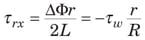In Equation (1), Φ = P + ρ gz. The volumetric flowrate Q can be related to the local shear rate by doing an integration by parts of Equation (2).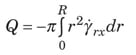Newtonian fluid. For a Newtonian fluid, τ rx = µY rx, which gives the following volumetric flowrate, known as the Hagen-Poiseuille equation.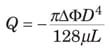It can be written in dimensionless form in Equation (4) with the two terms defined in Equations (5) and (6).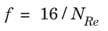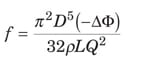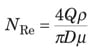Power law. A fluid that follows the power law model obeys the relationship τ rx = – µ(– Y rx) n. This gives the following equation.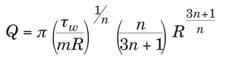Equation (7) can be rearranged into the following dimensionless form.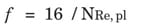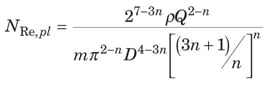Bingham plastic. In this case, there is a solid-like “plug flow” region from the pipe centerline (where τ rx = 0) to the point where – τ rx = τ 0 (that is, at r = r 0 = R x τ 0/ τ w). The result is a flow integral modified from Equation (2). For a Bingham plastic, – τ rx = τ 0 + µ ( Y rx). Using this expression and the modified flow integral, the Buckingham-Reiner Equation (10) is found.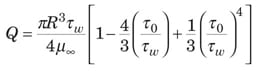The equivalent dimensionless form is given by Equations (11), (12) and (13).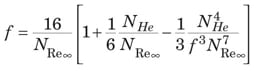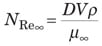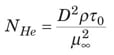## Turbulent Pipe Flow

Since most turbulent flows cannot be analyzed from a purely theoretical perspective, data and generalized dimensionless correlations are used.

Newtonian fluid. The friction factor for a Newtonian fluid in turbulent flow is a function of both N Re and the pipe relative roughness, ε /D, which can be read off the Moody diagram [ 5]. The turbulent part of the Moody diagram (for N Re > 4,000) is accurately represented by the Colebrook equation (14).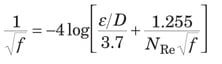When N Re is very large, the friction factor depends only on ε /D. This condition is noted with f T as the “fully turbulent” friction factor in Equation (15).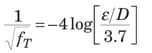The Churchill Equation [ 2] represents the entire Moody diagram, from laminar, through transition flow, to fully turbulent flow. It is presented here as Equations (16), (17), and (18).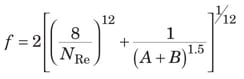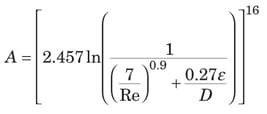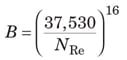Power law. For a power-law fluid, the friction factor depends only upon Equation (9) and the flow index, as represented by Equations (19) – (25) [ 3].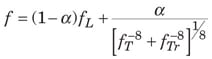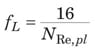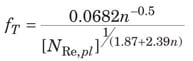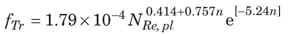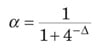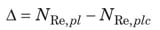The value of N Re where transition from laminar to turbulent flow occurs ( N Re,plc) is given by Equation (25).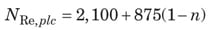Bingham plastic. For the Bingham plastic, f T is solely a function of N Re and N He, as represented by Equations (26) – (29).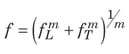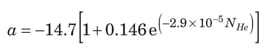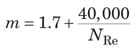## Definitions

Newtonian fluid. A fluid is known to be Newtonian when shear stresses associated with flow are directly proportional to the shear rate of the fluid

Power law fluid. A structural fluid has a structure that forms in the undeformed state, but then breaks down as shear rate increases. Such a fluid exhibits “power law” behavior at intermediate shear rates

Bingham plastic fluid. A plastic is a material that exhibits a yield stress, meaning that it behaves as a solid below the stress level and as a fluid above the stress level

## Nomenclature

a Dimensionless parameter

A Dimensionless parameter

B Dimensionless parameter

D Diameter, m

f Fanning friction factor, dimensionless

f L Laminar friction factor, dimensionless

f T Fully turbulent friction factor, dimensionless

f Tr Transition friction factor, dimensionless

g Gravitational acceleration, m/s 2

L Length of cylinder or pipe, m

m Consistency coefficient, (N)(s)/m 2

n Power law fluid flow index, dimensionless

N He Hedstrom number, dimensionless

N Re Reynolds Number, dimensionless

N Re,pl Power law Reynolds Number, dimensionless

N Re,plc Power law Reynolds Number at transition from laminar to turbulent flow, dimensionless

N Re∞ Bingham-plastic Reynolds Number, dimensionless

P Pressure, Pa

Q Volumetric flowrate, m 3 /s

r Radial position in a pipe or a cylinder, m

R Pipe or cylinder radius, m

V Velocity, m/s

z Vertical elevation above a horizontal reference plane, m

α Dimensionless parameter

Y rx Shear rate in tube flow, s – 1

ε Wall roughness, m

µ Newtonian viscosity, Pa – s

µ Bingham Plastic limiting viscosity, Pa – s

ρ Density, kg/m 3

τ 0 Yield stress, N/m 2

τ rx Stress due to force in x direction acting on r surface, N/m 2

τ w Stress exerted by fluid on tube wall, N/m 2

Φ Flow potential, P + ρ gz, Pa

âˆ†Φ Increase in flow potential, Pa

## References

1. Darby, R., Take the Mystery Out of Non-Newtonian Fluids, Chem. Eng., March 2001, pp. 66 – 73.

2. Churchil, S. W., Friction Factor Equation Spans all Fluid-Flow Regimes, Chem. Eng., November 1997, p. 91.

3. Darby, R., and Chang, H. D., A Generalized Correlation for Friction Loss in Drag-reducing Polymer Solutions, AIChE J., 30, p. 274, 1984.

4. Darby, R., and Chang, H. D., A Friction Factor Equation for Bingham Plastics, Slurries and Suspensions for all Fluid Flow Regimes, Chem. Eng., December 28, 1981, pp. 59 – 61.

5. Darby, R., “Fluid Mechanics for Chemical Engineers,” Vol. 2, Marcel Dekker, New York, N.Y., 2001.